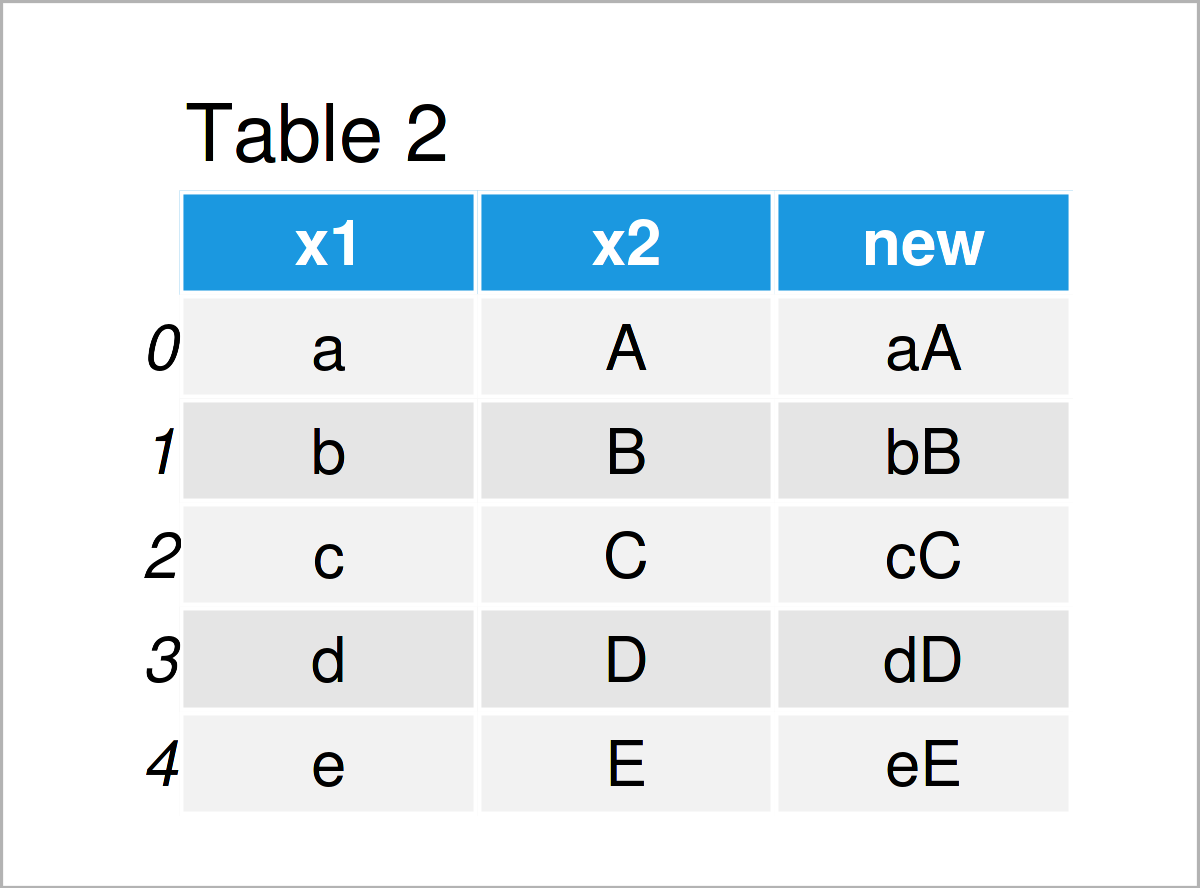# Combine Two Text Columns of pandas DataFrame in Python (Example)

In this Python article you’ll learn how to join text in columns of a pandas DataFrame.

The article will consist of these contents:

Here’s how to do it:

## Example Data & Libraries

We first need to import the pandas library, to be able to use the functions and commands that are contained in the library:

`import pandas as pd # Load pandas`

The following data is used as basement for this Python programming language tutorial:

```data = pd.DataFrame({'x1':['a', 'b', 'c', 'd', 'e'], # Create example DataFrame 'x2':['A', 'B', 'C', 'D', 'E']}) print(data) # Print example DataFrame```As you can see based on Table 1, our example data is a DataFrame and is made of five rows and the two variables “x1” and “x2”. Both variables contain text, i.e. alphabetical letters.

## Example: Join Two Text Columns as New DataFrame Variable

In this example, I’ll illustrate how to combine two pandas DataFrame variables in a new column.

For this, we can use the + sign as shown below:

```data_new = data.copy() # Create copy of DataFrame data_new['new'] = data_new['x1'] + data_new['x2'] # Concatenate columns print(data_new) # Print updated DataFrame```As shown in Table 2, the previous Python programming code has created a new pandas DataFrame object containing three columns. The first two columns are our input data and the third column is a combination of our two input variables x1 and x2.

## Video & Further Resources

If you need further info on the content of this tutorial, I can recommend watching the following video on the YouTube channel of Joe James. He shows further examples for the combination of multiple pandas DataFrame variables.

Please accept YouTube cookies to play this video. By accepting you will be accessing content from YouTube, a service provided by an external third party.If you accept this notice, your choice will be saved and the page will refresh.

Also, you could have a look at the related articles on this website:

At this point you should know how to merge text in columns of a pandas DataFrame in the Python programming language. Don’t hesitate to let me know in the comments, in case you have further questions.

Subscribe to the Statistics Globe Newsletter

Get regular updates on the latest tutorials, offers & news at Statistics Globe.
I hate spam & you may opt out anytime: Privacy Policy.